# The First Law of Thermodynamics

The First Law of Thermodynamics is one of the most important laws in all of science. Unfortunately, it is also one of the most confusing laws in all of science, and it tends to stump high school and college students. In this article, I will try to alleviate the confusion a little.

The First Law of Thermodynamics states that the change in a system’s internal energy is determined by two processes: heat and thermodynamic work. Mathematically, it is written as

where dU is the change in the system’s internal energy, δQ is the heat added to the system, and δA is the thermodynamic work done on the system.

But there’s so much to unpack in that little equation. Let’s take a step back and examine each of the concepts that underlie it. I’ll start with the concept of a system.

I. What is a system?

In physics, a “system” just means a thing. And it could be anything. Any collection of matter. Any group of particles. A house is a system. A car is a system. The grass in my backyard is a system. The air in this room is a system. A grain of sand is a system. And the entire universe is a system.

Systems are classified as being either open systems or closed systems. A closed system is a fixed group of particles; it’s always the same particles. In contrast, an open system is one in which we allow matter to enter and leave the system. For example, if we’re talking about the air in my lungs, that’s an open system because I’m breathing, which means air is constantly entering and leaving. In contrast, if we’re talking about the air in my lungs right this second, then that would be a closed system, because we’re referring to this specific collection of molecules. And as soon as I exhale, that closed system leaves my body and enters the atmosphere.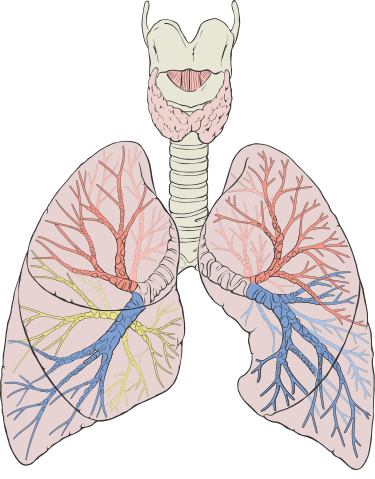The air in my lungs is an open system. But the air in my lungs right this second is a closed system, and as soon as I exhale, that closed system escapes into the atmosphere.

In thermodynamics, closed systems are much easier to think about than open systems. For the remainder of this article, whenever I say “system”, I mean closed system.

II. Three Kinds of Energy

Every system contains a certain amount of energy. There is no way to define the word “energy”; it’s just one of the fundamental concepts of science. But we can divide energy into three types: kinetic, potential, and internal.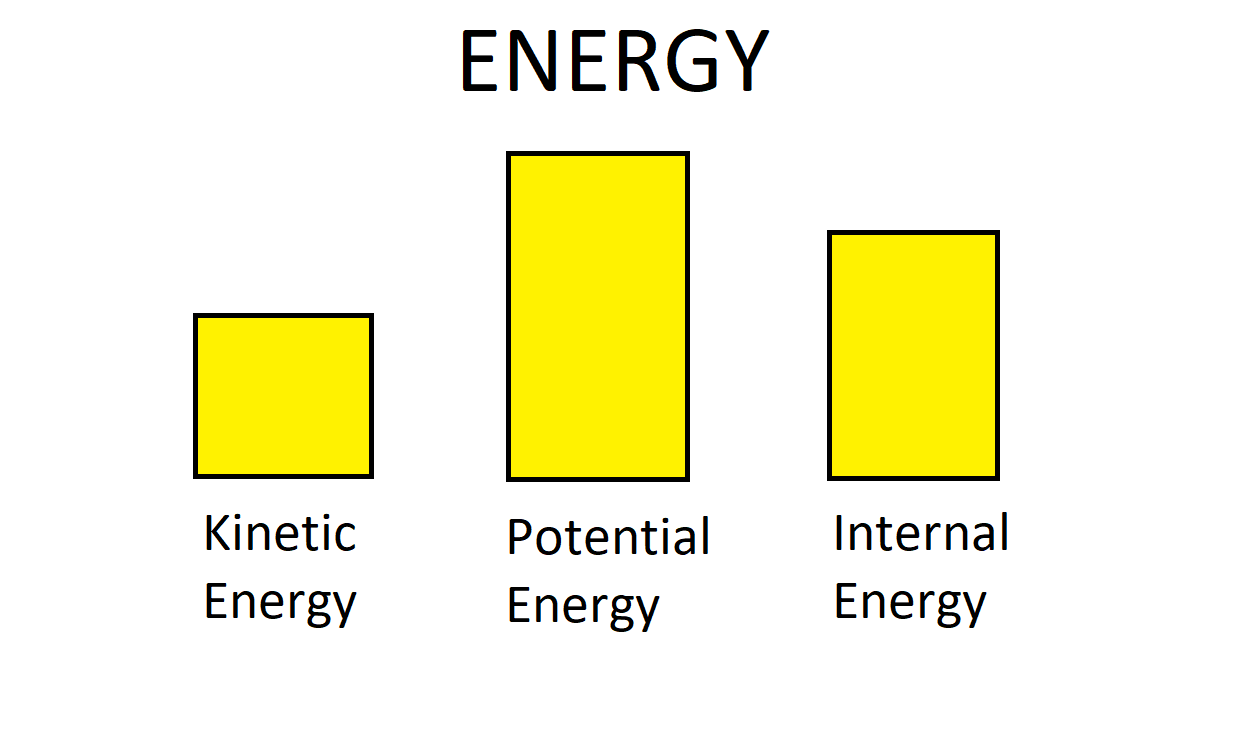A system possesses a certain amount of kinetic energy, a certain amount of potential energy, and a certain amount of internal energy. The sum of these three is the total energy of the system.

Kinetic energy (KE) is the energy of motion. For a single particle, it’s given by (1/2)mv^2, where m is the particle’s mass and v is its speed. For a system, we just add up the KE of each particle to get the KE of the entire system.Lots of kinetic energy in this picture. Each bird is flying.

Potential energy (PE) is the energy associated with the configuration of the particles in a system. There is PE associated with every conservative force that acts on a system. PE is defined according to the equation ΔPE = -W, where W is the work done by the force (see below). The most famous kind of potential energy is gravitational potential energy. Objects that are higher up from the ground have more gravitational potential energy, because they have farther to fall. In general, potential energy can be thought of as how much potential there is for an object to move. Something that’s higher up has the potential to fall a farther distance than something that’s lower down, and so it has more potential energy.The spire at the top of the Empire State Building has a large amount of potential energy, because it’s so high up from the ground.

Out of the three kinds of energy, internal energy (IE) is the hardest one to define. Personally, I like to think of internal energy as being the energy contained within molecules, or among molecules. KE and PE are both defined on a macroscopic level; that is, they are bulk terms, and you don’t have to worry about microscopic things like atoms and molecules. In contrast, IE is defined on a microscopic level. It is the energy contained within and among molecules. For a single molecule, such an energy would be infinitesimally small, but there are lots and lots of molecules in a system, such that it adds up to a significant quantity of energy.

I also like to think of internal energy as being non-Newtonian energy, because it involves concepts that Newtonian physics could never account for. In Isaac Newton’s day, they didn’t know about molecules or microscopic forces. KE and PE are both staples of Newtonian physics, whereas IE was not fully understood until the 19th Century (long after Newton’s time).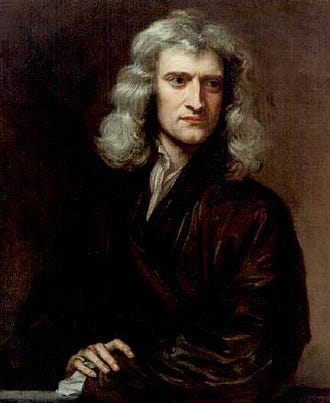Isaac Newton never could have understood internal energy, because it involves concepts which were not discovered until long after his time.

Internal energy is often denoted by the letter U, as it is in the First Law of Thermodynamics.

Every system contains a certain amount of KE, a certain amount of PE, and a certain amount of IE. These three quantities add up to the total energy of the system. But the First Law of Thermodynamics (which I will abbreviate as the 1st Law of TD) concerns only the internal energy of a system. The 1st Law of TD tells us how the IE of a system will change over time.

III. Transfer of Energy

There are two ways of transferring energy to a system: work and heat. Work is when you exert a force on a system and displace some (or all) of the particles in that system. By doing so, you have transferred energy to (or from) the system. Work is subdivided into two kinds: mechanical work and thermodynamic work (see Part IV, below).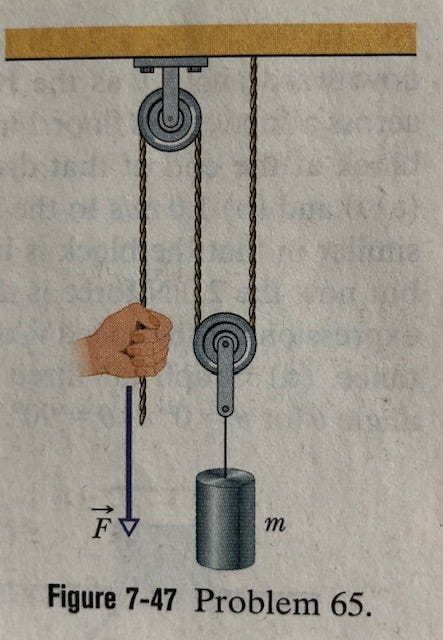Work is when you exert a force on a system and you make it move in some way. (Picture from Halliday, Resnick, & Walker, 2014)

Meanwhile, heat is when one system transfers energy to another by means of conduction (a warm object warms an adjacent cool object), radiation (like how the sun warms the earth), or latent heat release (like when water vapor condenses to form a cloud). The weird thing about heat is that in science, “heat” is a verb, not a noun. Heat is not a kind of energy; it is a means of transferring energy.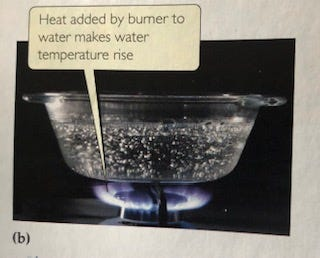The flame heats the water. (Picture from Brown et al., 2015)

Heat should not be confused with temperature; in science, these are two distinct concepts. It is true that when you heat a system, its temperature usually (but not always) rises. But that doesn’t mean that heat is temperature. Temperature is a quantity that measures the average speed of a system’s particles; heat, meanwhile, is a process. Heat is one of two ways of transferring energy to a system, work being the other.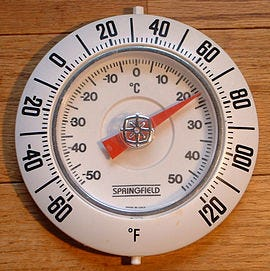Heat and temperature are not the same thing. Temperature is a quantity, whereas heat is a process.

IV. Two Kinds of Work

There are two kinds of work: mechanical work and thermodynamic work. The best way to explain the difference would just be to provide some examples:

If you kick a ball across a field, that’s mechanical work. When you kicked the ball, you transferred energy to it, which is why the ball gained so much kinetic energy.When you kick a ball, you do mechanical work on it. You transfer kinetic energy to the ball, which is why it moves.

If a piston pushes down on the air inside a cylinder, that’s thermodynamic work. By compressing it, the piston transferred energy to the air in the cylinder, and as a result, that air should become warmer. (To be fair, the piston also did a small amount of mechanical work on the air, just by pushing on it.)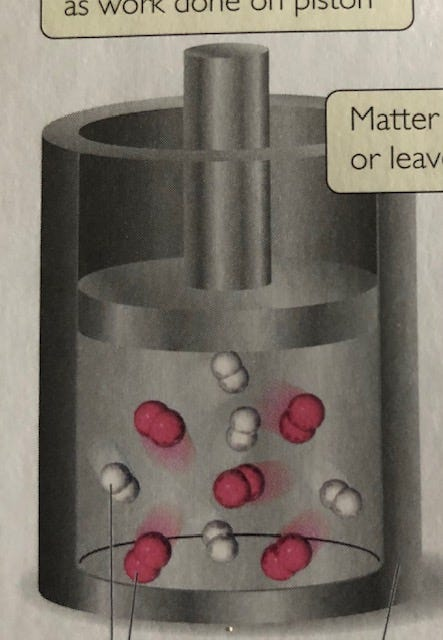When the piston compresses the air in the cylinder, it does thermodynamic work on it. As such, that air should become warmer. (Picture from Brown et al., 2015)

If you push a box across a floor, that’s mechanical work. You transferred energy to the box, allowing it to move across the floor.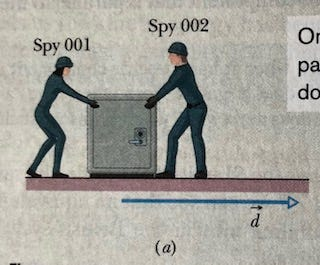When you push a box across the floor, you performed mechanical work on it. You transferred kinetic energy to it. (Picture from Halliday, Resnick, & Walker, 2014)

If a mass of air rises in the atmosphere, and cools as it rises, that’s thermodynamic work. Because the air is moving upwards into an area where the density is lower, the mass of air expands. By expanding, it performs work on its surroundings, and therefore, it loses energy. That’s why the air mass cools as it rises. And because it’s cooling, some of its water vapor might condense into droplets of liquid water — that’s how clouds form.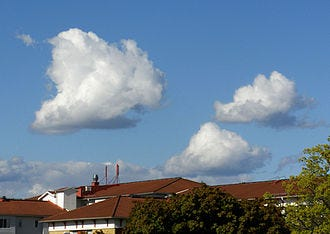Clouds form when a rising air mass cools. It cools because it expanded, which means that it did thermodynamic work on its surroundings.

Essentially, the difference between the two kinds of work is that mechanical work involves moving an entire object without deforming it, whereas thermodynamic work involves changing the volume or the arrangement of a system, but without necessarily moving the whole thing. If you make something move, that’s mechanical work; if you stretch or compress something, that’s thermodynamic work.

V. The First Law of Thermodynamics

Finally, we return to the 1st Law of TD. Recall that the 1st Law of TD states that

where dU is the change in internal energy, δQ is the heat added, and δA is the thermodynamic work done.

The First Law of Thermodynamics states that for any system, over an infinitesimal interval of time (dt), the change in the system’s internal energy (dU) is equal to the heat added to the system (δQ) plus the thermodynamic work done on the system (δA). In other words, heat and (thermodynamic) work are the two processes that contribute to a system’s internal energy.

The reason all three of these quantities are denoted with d’s (or δ’s) is that they are differential quantities: it’s assumed that we are only observing what happens to this system over an infinitesimally small interval of time, dt. However, we could integrate it over an actual, nonzero interval of time, and then we could lose the d’s: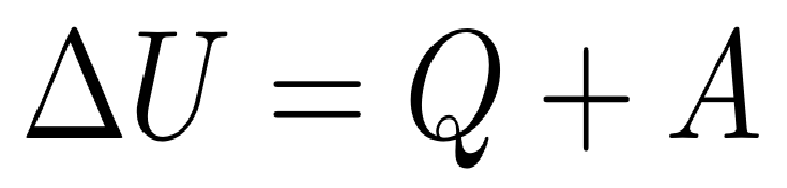The 1st Law of TD over a nonzero interval of time

where ΔU is the change in internal energy, Q is the heat added, and A is the thermodynamic work done.

Meanwhile, the reason we write the heating and work terms as δQ and δA, rather than dQ and dA, is because they are not state functions. Internal energy is a state function, but work and heat are not. That’s why we write the change in internal energy as dU, but write the heating and work terms as δQ and δA. A state function is a property of a system in the present, which you could measure without having to know about what was happening to the system beforehand.

The significance of the 1st Law of TD is that it tells us what happens to a system’s internal energy. It tells us that there are two ways to increase a system’s internal energy: you can heat it or you can perform thermodynamic work on it. Over a period of time, the change in a system’s internal energy is equal to the heat added to the system over that period plus the thermodynamic work done on the system over that period.

The 1st Law of TD also highlights the difference between mechanical work and thermodynamic work: mechanical work changes the KE and the PE of a system, whereas thermodynamic work — along with heat — changes its IE. Thermodynamic work is work that changes a system’s internal energy.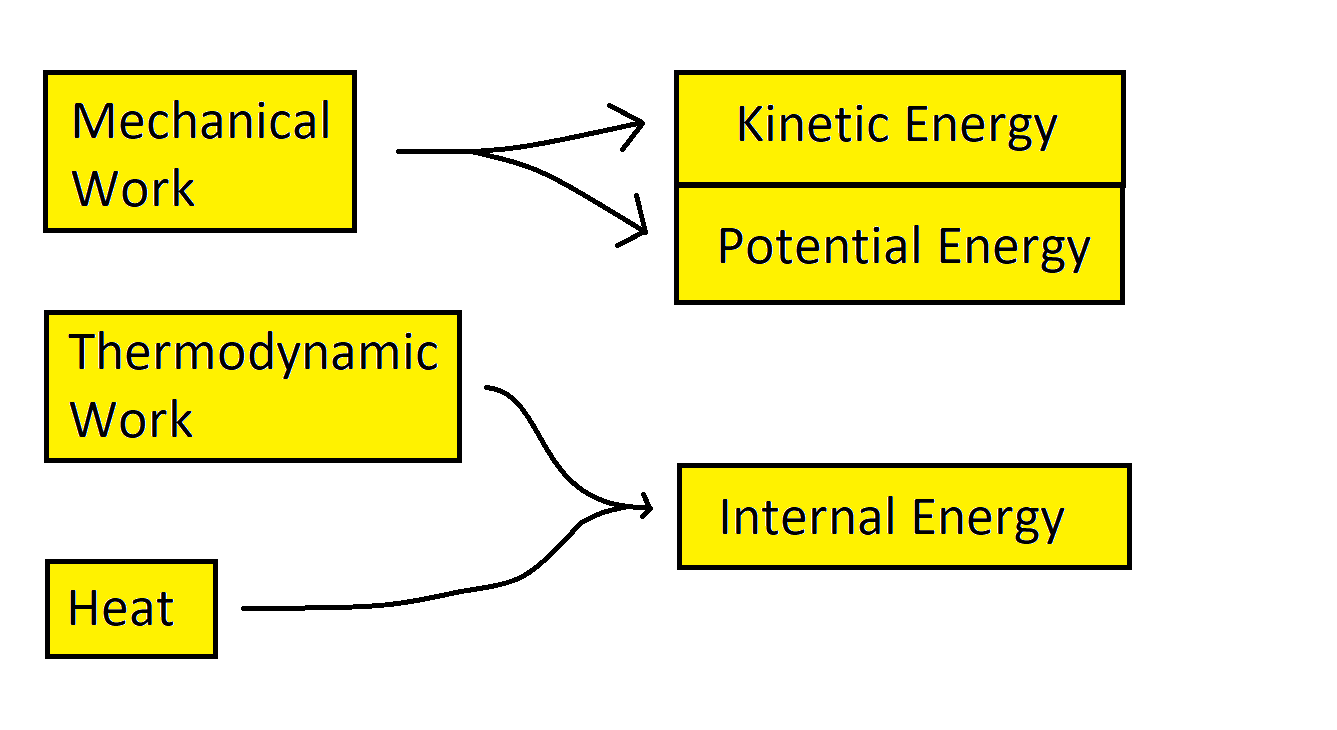Mechanical work adds to the kinetic and potential energies of a system, whereas thermodynamic work — along with heat — adds to its internal energy.

VI. Pressure-volume Work

In many contexts, we can make a further simplification to the 1st Law of TD. We can assume that the only thermodynamic work that was performed on a system was pressure-volume work, which is the work involved in the expansion or compression of gases. If we further assume that pressure is constant (and in many contexts, it is), then there’s a very easy way to compute the pressure-volume work: W = -pΔV, where W is the pressure-volume work done on a system, p is the system’s pressure, and V is the system’s volume (which changed). Therefore, under these assumptions, we can rewrite the 1st Law of TD as: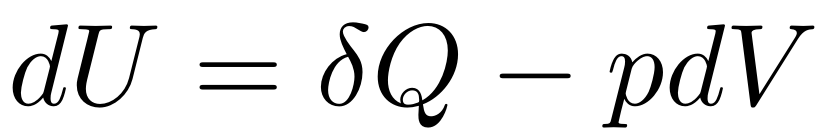The 1st Law of TD, under the assumptions of only pressure-volume work and constant pressure

And this is the form of the 1st Law of TD that you’ll find in some textbooks. Furthermore, by combining this with another quantity called enthalpy (H), we can again rewrite the 1st Law of TD as

Enthalpy is defined by H = U + pV. But enthalpy is beyond the scope of this article.

VII. The 1st Law of TD and the LCE

Many introductory-level science textbooks will tell you that the First Law of Thermodynamics is the statement that energy cannot be created or destroyed; it can only be transferred from one system to another, or converted from one form to another. But they’re wrong. That is the Law of Conservation of Energy (LCE), not the First Law of Thermodynamics. These two laws are related to each other, but they’re not the same thing.

The Law of Conservation of Energy states that the total amount of energy in the universe is a constant. Energy can never be created or destroyed; it can only be transferred from one system to another or converted from one form to another (e. g. converted from KE to PE). Because of this, if you have an isolated system — a system that has no interactions with any other system — then that system’s total energy is a constant. It can never change.

In contrast, the 1st Law of TD is much more specific. Instead of telling us about total energy, it tells us about internal energy, and it outlines the two processes by which a system’s internal energy can be changed: heat and thermodynamic work.

To be fair, the two laws are related to each other. Both laws describe how a system’s energy changes over time. And in some ways, you could say the the 1st Law of TD is just a version of the LCE: it’s the version where we only consider internal energy. Nevertheless, the First Law of Thermodynamics and the Law of Conservation of Energy are not the same law, and I wish science textbooks would stop pretending that they are. They’re not.

VIII. Works Cited:

1. Atmospheric Thermodynamics (Second Edition), by Iribarne & Godson, 1981.

2. Chemistry: The Central Science (13th Edition), by Brown et al. 2015.

3. Fundamentals of Physics: Tenth Edition, by Halliday, Resnick, & Walker, 2014.

4. Wikipedia.